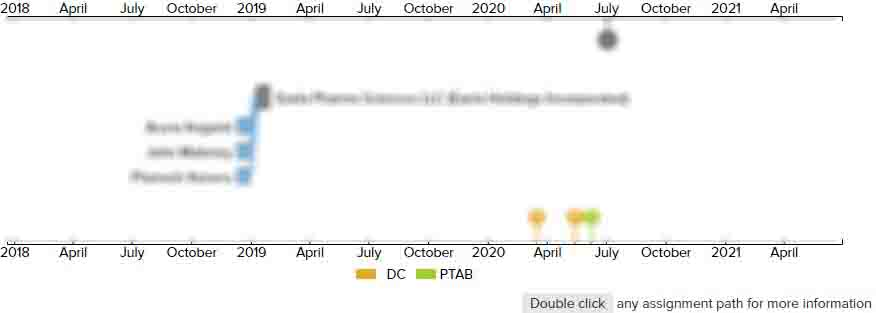Data analysis methods for locating entities of interest within large, multivariable datasets

• US 8,131,473 B1
• Filed: 05/20/2005
• Issued: 03/06/2012
• Est. Priority Date: 05/20/2004
• Status: Active Grant
First Claim
Patent Images

1. A method for locating a subset within an experimental set of biological data points that is of most interest for further analysis, the method comprising:

• a) obtaining a set of data points associated with a biological phenomenon of interest, wherein the set of data points comprises a baseline and one or more experimental groups;

b) designating a trend of interest in the set of data points that is associated with the biological phenomenon of interest, wherein the trend indicates a relationship between the data points and an independent variable,c) developing a mathematical model of the trend, the mathematical model being a function of the independent variable and wherein the mathematical model models the trend with respect to the independent variable;

d) testing each data point in the set for adherence to the mathematical model, wherein the data points adhering to the model are identified as being members of the subset of most interest for further analysis; and

e) providing identification of the members of the subset in a user-readable format,wherein all of the steps b), c), d), and e) are performed on a suitably-programmed computer.

• 9 Assignments
Timeline View
Assignment View×
×